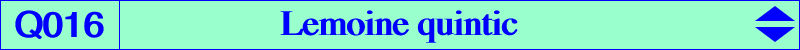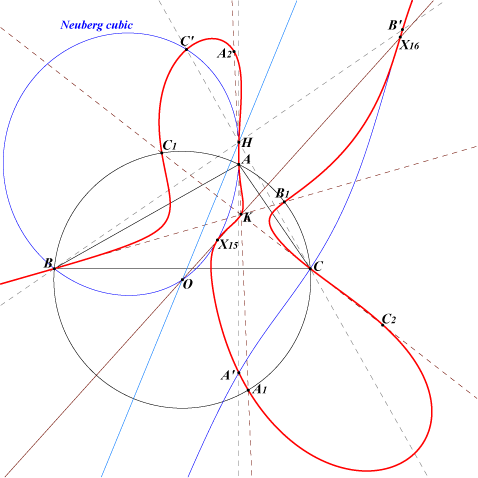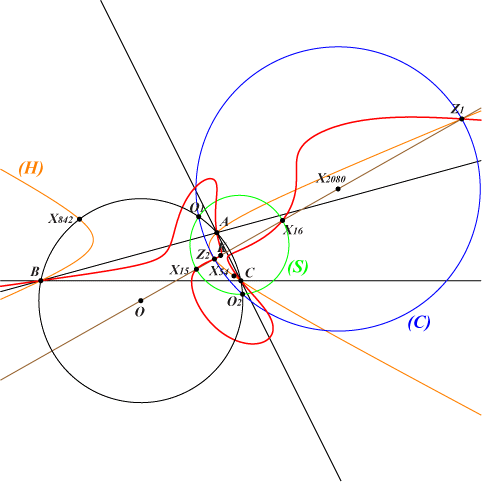too complicated to be written here. Click on the link to download a text file.X(4), X(6), X(15), X(16), X(524) A', B', C' : reflections of A, B, C in the sidelines of ABC A1, B1, C1 : second common points of the symmedians and the circumcircle A2 homothetic of A1 under h(A,-1/2), B2, C2 similarly foci of the inscribed Steiner ellipse. Z1, Z2 : see belowK = X(6) is the Lemoine point of triangle ABC. For any point P, denote by Ka the Lemoine point of triangle PBC and define Kb, Kc similarly. Triangles ABC and KaKbKc are perspective if and only if P lies on the Lemoine quintic Q016 (adapted from a Hyacinthos message by Jean-Pierre Ehrmann). The locus of the perspector is Q156. Q016 is a bicircular quintic with a real asymptote parallel to the line GK. The tangents at A, B, C pass through K and those at A1, B1, C1 pass through O. The tangents at the isodynamic points X(15), X(16) are those of the Neuberg cubic. Thus, we know the 15 common points of Q016 and the Neuberg cubic. See the analogous Q139. *** Two remarkable points on Q016 Q016 meets the Brocard axis at X(6), X(15), X(16) and two remaining points Z1 = X(39229), Z2 = X(39230).These points Z1, Z2 • lie on on the circum-conic (H) passing through X(i) for these i : {54, 842, 7608, 10415, 10562, 14536, 23061, 27867, 34110}, perspector X(39232), center X(39233). • are the end points of a diameter of the circle (C) with center X(2080), a member of the pencil of coaxal circles conaining the circumcircle (O), the Schoute circle (S), whose radical axis is perpendicular at K to the Brocard axis. (C) is orthogonal to the circles with diameters {3, 576}, {6, 574}, {39, 5038}, {182, 32447}, {187, 8586}. • lie on the cubics K273, K303a, K303b, K305, K800, K854. • have a simple barycentric product Z12 = X(39231), on the lines {50, 237}, {160, 184}, {187, 2393}, {524, 2080} and many others.